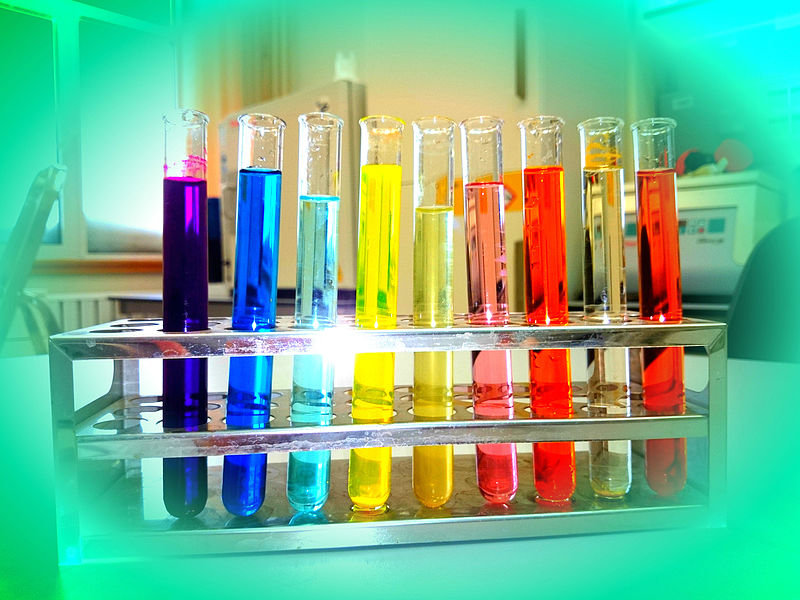# Difference Between Ideal Solution and Non ideal Solution

## Main Difference – Ideal Solution Vs Non ideal Solution

An ideal solution is a solution that has similar properties to an ideal mixture of gases. However, there are no interactions between gas molecules in ideal gases. But we cannot consider the same in solutions because molecules in solutions should have intermolecular interactions between molecules in order to be considered as a liquid. Therefore, ideal solutions differ from ideal gases due to the presence of interactions between molecules. Non ideal solutions are the actual solutions present everywhere. But there are some solutions that have a similar behavior to an ideal solution. The main difference between ideal solution and non ideal solution is that the intermolecular interactions between all the molecules are the same in ideal solutions whereas the intermolecular interactions between solute molecules and solvent molecules are different from each other in non ideal solutions.

### Key Areas Covered

1. What is an Ideal Solution
– Characteristic Properties and Existence
2. What is a Non Ideal Solution
– Properties and Examples
3. What is the Difference Between Ideal Solution and Non Ideal Solution
– Comparison of Key Differences

Key Terms: Ideal Solution, Intermolecular Interactions, Non ideal Solution, Solution, Van Der Waal Forces## What is an Ideal Solution

An ideal solution is a solution in which interactions between molecules are identical between all molecules in the solution. There is no net force between components of the solution. Thus, the distance between molecules of a solute does not change after mixing it with a solvent. This is because there should be a force acting on each and every molecule of the solute compound in order to change the distance.

The enthalpy change of an ideal solution is zero or approximately equal to zero. This means the enthalpy of initial components is equal to the enthalpy of the solution after mixing. Therefore, the enthalpy for missing is zero.Almost ideal solutions exist in reality. For example, the mixture of benzene and toluene forms almost an ideal solution. Here, the interactions between benzene-benzene, benzene-toluene and toluene-toluene are almost identical.

## What is a Non ideal Solution

A non ideal solution is a solution that has differences in the interactions between molecules of different components in the solution. A non ideal solution can be recognized by determining the strength of the intermolecular forces. The properties of a non ideal solution may depend on the solvent-solvent, solvent-solute and the solute-solute interactions. If the solvent-solute interactions are higher than other two types of interactions, then the solute is well soluble in that solvent. The resulting mixture is a non ideal solution.

However, highly dilute solutions show ideal solution behavior more than non ideal solution behavior. This is because, the amount of the solute molecules in a dilute solution is less resulting in a less attraction between solute molecules. But in concentrated solutions, there are more solute molecules. Then there are stronger interactions between solute molecules. Concentrated solutions show non ideal solution behavior.

The mixing enthalpy change of solutes with the solvent is a higher value. This means the initial enthalpy of solutes and solvent is either very high or very lower than the enthalpy of the final solution.Figure 2: How Raoult’s law work on ideal and non ideal solutions

The above image shows Raoult’s deviation pressure diagram. As shown in the diagram, there can be two types of non ideal solutions: solutions that show a negative deviation from ideal solutions and solutions show positive deviation from the ideal solutions. The negative deviation is caused when solute molecules are more attracted to each other than they are attracted by the solvent molecules. A positive deviation occurs when the solute-solvent attractions are higher than the attractions between similar molecules.

## Difference Between Ideal Solution and Non ideal Solution

### Definition

Ideal Solution: An ideal solution is a solution where interactions between molecules are identical between all the molecules in the solution.

Non ideal Solution: A non ideal solution is a solution that has differences in the interactions between molecules of different components in the solution.

### Types of Interactions

Ideal Solution: Ideal solutions have identical interactions between all the molecules of all components.

Non ideal Solution: Non ideal solutions have solvent-solvent, solvent-solute and solute-solute interactions.

### Enthalpy

Ideal Solution: The change in enthalpy when an ideal solution forms is zero or approximately zero.

Non ideal Solution: The change in enthalpy when a non ideal solution forms is either a positive or negative value.

### Real Solutions

Ideal Solution: Highly dilute solutions can behave as ideal solutions.

Non ideal Solution: Concentrated solutions behave as non ideal solutions.

### Conclusion

Although there are no ideal gases, ideal solutions may exist in reality. Highly diluted solution also behave as ideal solutions due to less interactions between solute molecules and solute-solvent molecules. The main difference between ideal solution and non ideal solution is that the intermolecular interactions between all the molecules are the same in ideal solutions whereas the intermolecular interactions between solute molecules and solvent molecules are different from each other in non ideal solutions.

##### References:

1.“Ideal solution.” Encyclopædia Britannica, Encyclopædia Britannica, inc., Available here. Accessed 17 Aug. 2017.
2.“Introduction to Non ideal Solutions.” Chemistry LibreTexts, Libretexts, 9 Jan. 2017, Available here. Accessed 17 Aug. 2017.

##### Image Courtesy:

1. “Chemical solutions” By Bismoldirs – Own work (CC BY-SA 4.0) via Commons Wikimedia
2. “RaoultDeviationPressureDiagram” By Karlhahn at English Wikipedia – Transferred from en.wikipedia to Commons. (Public Domain) via Commons Wikimedia